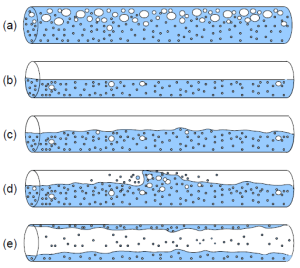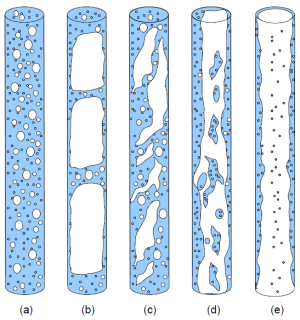# Package Modelica.​Fluid.​Dissipation.​PressureLoss.​StraightPipePackage for pressure loss calculation of straight pipes

### Information

#### Straight Pipe

##### Laminar flow

Calculation of pressure loss in a straight pipe for laminar flow regime of single-phase fluid flow only. See more information.

##### Turbulent flow

Calculation of pressure loss in a straight pipe for turbulent flow regime of single-phase fluid flow only considering surface roughness. See more information.

##### Overall flow

Calculation of pressure loss in a straight pipe for laminar or turbulent flow regime of single-phase fluid flow only considering surface roughness. See more information.

##### Two phase overall flow

Calculation of pressure loss for two phase flow in a horizontal or vertical straight pipe for an overall flow regime considering frictional, momentum and geodetic pressure loss. See more information.

Extends from `Modelica.​Icons.​VariantsPackage` (Icon for package containing variants).

### Package Contents

NameDescription
`dp_laminar_DP`Pressure loss of straight pipe | calculate pressure loss| laminar flow regime (Hagen-Poiseuille)
`dp_laminar_IN_con`Input record for function dp_laminar_DP and dp_laminar_MFLOW
`dp_laminar_IN_var`Input record for function dp_laminar_DP and dp_laminar_MFLOW
`dp_laminar_MFLOW`Pressure loss of straight pipe | calculate mass flow rate | laminar flow regime (Hagen-Poiseuille)
`dp_overall_DP`Pressure loss of straight pipe | calculate pressure loss | overall flow regime | surface roughness
`dp_overall_IN_con`Input record for function dp_overall_DP and dp_overall_MFLOW
`dp_overall_IN_var`Input record for function dp_overall_DP and dp_overall_MFLOW
`dp_overall_MFLOW`Pressure loss of straight pipe | calculate mass flow rate | overall flow regime | surface roughness
`dp_turbulent_DP`Pressure loss of straight pipe | calculate pressure loss | turbulent flow regime | surface roughness
`dp_turbulent_IN_con`Input record for function dp_turbulent_DP and dp_turbulent_MFLOW
`dp_turbulent_IN_var`Input record for function dp_turbulent_DP and dp_turbulent_MFLOW
`dp_turbulent_MFLOW`Pressure loss of straight pipe | calculate mass flow rate | turbulent flow regime | surface roughness
`dp_twoPhaseOverall_DP`Pressure loss of straight pipe for two phase flow | calculate (frictional, momentum, geodetic) pressure loss
`dp_twoPhaseOverall_IN_con`Input record for function dp_twoPhaseOverall_DP
`dp_twoPhaseOverall_IN_var`Input record for function dp_twoPhaseOverall_DP

## Function Modelica.​Fluid.​Dissipation.​PressureLoss.​StraightPipe.​dp_laminar_DPPressure loss of straight pipe | calculate pressure loss| laminar flow regime (Hagen-Poiseuille)

### Information

Calculation of pressure loss in a straight pipe for laminar flow regime of an incompressible and single-phase fluid flow only.

Generally this function is numerically best used for the incompressible case , where the mass flow rate (m_flow) is known (as state variable) in the used model and the corresponding pressure loss (DP) has to be calculated. On the other hand the function dp_laminar_MFLOW is numerically best used for the compressible case if the pressure loss (dp) is known (out of pressures as state variable) and the mass flow rate (M_FLOW) has to be calculated. See more information.

Extends from `Modelica.​Icons.​Function` (Icon for functions).

### Inputs

TypeNameDescription
`dp_laminar_IN_con``IN_con`Input record for function dp_laminar_DP
`dp_laminar_IN_var``IN_var`Input record for function dp_laminar_DP
`MassFlowRate``m_flow`Mass flow rate

### Outputs

TypeNameDescription
`Pressure``DP`Output for function dp_laminar_DP

## Function Modelica.​Fluid.​Dissipation.​PressureLoss.​StraightPipe.​dp_laminar_MFLOWPressure loss of straight pipe | calculate mass flow rate | laminar flow regime (Hagen-Poiseuille)

### Information

Calculation of pressure loss in a straight pipe for laminar flow regime of an incompressible and single-phase fluid flow only.

Generally this function is numerically best used for the compressible case , where the pressure loss (dp) is known (out of pressures as state variable) in the used model and the corresponding mass flow rate (M_FLOW) has to be calculated. On the other hand the function dp_laminar_DP is numerically best used for the incompressible case if the mass flow rate (m_flow) is known (as state variable) and the pressure loss (DP) has to be calculated. See more information.

Extends from `Modelica.​Icons.​Function` (Icon for functions).

### Inputs

TypeNameDescription
`dp_laminar_IN_con``IN_con`Input record for function dp_laminar_MFLOW
`dp_laminar_IN_var``IN_var`Input record for function dp_laminar_MFLOW
`Pressure``dp`Pressure loss

### Outputs

TypeNameDescription
`MassFlowRate``M_FLOW`Output for function dp_laminar_MFLOW

## Record Modelica.​Fluid.​Dissipation.​PressureLoss.​StraightPipe.​dp_laminar_IN_conInput record for function dp_laminar_DP and dp_laminar_MFLOW

### Information

This record is used as input record for the pressure loss function dp_laminar_DP and dp_laminar_MFLOW.

Extends from `Modelica.​Fluid.​Dissipation.​Utilities.​Records.​PressureLoss.​StraightPipe` (Input for straight pipe).

### Fields

TypeNameDescription
`Diameter``d_hyd`Hydraulic diameter
`Length``L`Length

## Record Modelica.​Fluid.​Dissipation.​PressureLoss.​StraightPipe.​dp_laminar_IN_varInput record for function dp_laminar_DP and dp_laminar_MFLOW

### Information

This record is used as input record for the pressure loss function dp_laminar_DP and dp_laminar_MFLOW.

Extends from `Modelica.​Fluid.​Dissipation.​PressureLoss.​StraightPipe.​dp_overall_IN_var` (Input record for function dp_overall_DP and dp_overall_MFLOW).

### Fields

TypeNameDescription
`DynamicViscosity``eta`Dynamic viscosity of fluid
`Density``rho`Density of fluid

## Function Modelica.​Fluid.​Dissipation.​PressureLoss.​StraightPipe.​dp_overall_DPPressure loss of straight pipe | calculate pressure loss | overall flow regime | surface roughness

### Information

Calculation of pressure loss in a straight pipe for overall flow regime of an incompressible and single-phase fluid flow only considering surface roughness.

Generally this function is numerically best used for the incompressible case , where the mass flow rate (m_flow) is known (as state variable) in the used model and the corresponding pressure loss (DP) has to be calculated. On the other hand the function dp_overall_MFLOW is numerically best used for the compressible case if the pressure loss (dp) is known (out of pressures as state variable) and the mass flow rate (M_FLOW) has to be calculated. See more information.

Extends from `Modelica.​Icons.​Function` (Icon for functions).

### Inputs

TypeNameDescription
`dp_overall_IN_con``IN_con`Input record for function dp_overall_DP
`dp_overall_IN_var``IN_var`Input record for function dp_overall_DP
`MassFlowRate``m_flow`Mass flow rate

### Outputs

TypeNameDescription
`Pressure``DP`Output for function dp_overall_DP

## Function Modelica.​Fluid.​Dissipation.​PressureLoss.​StraightPipe.​dp_overall_MFLOWPressure loss of straight pipe | calculate mass flow rate | overall flow regime | surface roughness

### Information

Calculation of pressure loss in a straight pipe for overall flow regime of an incompressible and single-phase fluid flow only considering surface roughness.

Generally this function is numerically best used for the compressible case , where the pressure loss (dp) is known (out of pressures as state variable) in the used model and the corresponding mass flow rate (M_FLOW) has to be calculated. On the other hand the function dp_overall_DP is numerically best used for the incompressible case if the mass flow rate (m_flow) is known (as state variable) and the pressure loss (DP) has to be calculated. See more information.

Extends from `Modelica.​Icons.​Function` (Icon for functions).

### Inputs

TypeNameDescription
`dp_overall_IN_con``IN_con`Input record for function dp_overall_MFLOW
`dp_overall_IN_var``IN_var`Input record for function dp_overall_MFLOW
`Pressure``dp`Pressure loss

### Outputs

TypeNameDescription
`MassFlowRate``M_FLOW`Output of function dp_overall_MFLOW

## Record Modelica.​Fluid.​Dissipation.​PressureLoss.​StraightPipe.​dp_overall_IN_conInput record for function dp_overall_DP and dp_overall_MFLOW

### Information

This record is used as input record for the pressure loss function dp_overall_DP and dp_overall_MFLOW.

Extends from `Modelica.​Fluid.​Dissipation.​PressureLoss.​StraightPipe.​dp_turbulent_IN_con` (Input record for function dp_turbulent_DP and dp_turbulent_MFLOW).

### Fields

TypeNameDescription
`Roughness``roughness`Choice of considering surface roughness
`Diameter``d_hyd`Hydraulic diameter
`Length``L`Length
`Length``K`Roughness (average height of surface asperities)

## Record Modelica.​Fluid.​Dissipation.​PressureLoss.​StraightPipe.​dp_overall_IN_varInput record for function dp_overall_DP and dp_overall_MFLOW

### Information

This record is used as input record for the pressure loss function dp_overall_DP and dp_overall_MFLOW.

Extends from `Modelica.​Fluid.​Dissipation.​Utilities.​Records.​General.​PressureLoss` (Base record for fluid properties for pressure loss).

### Fields

TypeNameDescription
`DynamicViscosity``eta`Dynamic viscosity of fluid
`Density``rho`Density of fluid

## Function Modelica.​Fluid.​Dissipation.​PressureLoss.​StraightPipe.​dp_turbulent_DPPressure loss of straight pipe | calculate pressure loss | turbulent flow regime | surface roughness

### Information

Calculation of pressure loss in a straight pipe for turbulent flow regime of an incompressible and single-phase fluid flow only considering surface roughness.

Generally this function is numerically best used for the incompressible case , where the mass flow rate (m_flow) is known (as state variable) in the used model and the corresponding pressure loss (DP) has to be calculated. On the other hand the function dp_turbulent_MFLOW is numerically best used for the compressible case if the pressure loss (dp) is known (out of pressures as state variable) and the mass flow rate (M_FLOW) has to be calculated. See more information.

Extends from `Modelica.​Icons.​Function` (Icon for functions).

### Inputs

TypeNameDescription
`dp_turbulent_IN_con``IN_con`Input record for function dp_turbulent_DP
`dp_turbulent_IN_var``IN_var`Input record for function dp_turbulent_DP
`MassFlowRate``m_flow`Mass flow rate

### Outputs

TypeNameDescription
`Pressure``DP`Output for function dp_turbulent_DP

## Function Modelica.​Fluid.​Dissipation.​PressureLoss.​StraightPipe.​dp_turbulent_MFLOWPressure loss of straight pipe | calculate mass flow rate | turbulent flow regime | surface roughness

### Information

Calculation of pressure loss in a straight pipe for turbulent flow regime of an incompressible and single-phase fluid flow only considering surface roughness.

Generally this function is numerically best used for the compressible case if the pressure loss (dp) is known (out of pressures as state variable) and the mass flow rate (M_FLOW) has to be calculated. On the other hand the function dp_turbulent_DP is numerically best used for the incompressible case , where the mass flow rate (m_flow) is known (as state variable) in the used model and the corresponding pressure loss (DP) has to be calculated. See more information.

Extends from `Modelica.​Icons.​Function` (Icon for functions).

### Inputs

TypeNameDescription
`dp_turbulent_IN_con``IN_con`Input record for function dp_turbulent_MFLOW
`dp_turbulent_IN_var``IN_var`Input record for function dp_turbulent_MFLOW
`Pressure``dp`Pressure loss

### Outputs

TypeNameDescription
`MassFlowRate``M_FLOW`Mass flow rate

## Record Modelica.​Fluid.​Dissipation.​PressureLoss.​StraightPipe.​dp_turbulent_IN_conInput record for function dp_turbulent_DP and dp_turbulent_MFLOW

### Information

This record is used as input record for the pressure loss function dp_turbulent_DP and dp_turbulent_MFLOW.

Extends from `Modelica.​Fluid.​Dissipation.​Utilities.​Records.​PressureLoss.​StraightPipe` (Input for straight pipe).

### Fields

TypeNameDescription
`Roughness``roughness`Choice of considering surface roughness
`Diameter``d_hyd`Hydraulic diameter
`Length``L`Length
`Length``K`Roughness (average height of surface asperities)

## Record Modelica.​Fluid.​Dissipation.​PressureLoss.​StraightPipe.​dp_turbulent_IN_varInput record for function dp_turbulent_DP and dp_turbulent_MFLOW

### Information

This record is used as input record for the pressure loss function dp_turbulent_DP and dp_turbulent_MFLOW.

Extends from `Modelica.​Fluid.​Dissipation.​PressureLoss.​StraightPipe.​dp_overall_IN_var` (Input record for function dp_overall_DP and dp_overall_MFLOW).

### Fields

TypeNameDescription
`DynamicViscosity``eta`Dynamic viscosity of fluid
`Density``rho`Density of fluid

## Function Modelica.​Fluid.​Dissipation.​PressureLoss.​StraightPipe.​dp_twoPhaseOverall_DPPressure loss of straight pipe for two phase flow | calculate (frictional, momentum, geodetic) pressure loss

### Information

Calculation of pressure loss for two phase flow in a horizontal or vertical straight pipe for an overall flow regime considering frictional, momentum and geodetic pressure loss.

Generally the pressure loss for two phase flow in a horizontal or a vertical straight pipe can be calculated for the following fluid flow regimes:

Horizontal fluid flow [(a) bubble flow, (b) stratified flow, (c) wavy flow, (d) slug flow, (e) annular flow]:Vertical fluid flow [(a) bubble flow, (b) plug slug flow, (c) foam flow, (d) annular streak flow, (e) annular flow]:Extends from `Modelica.​Icons.​Function` (Icon for functions).

### Inputs

TypeNameDescription
`dp_twoPhaseOverall_IN_con``IN_con`Input record for function dp_twoPhaseOverall_DP
`dp_twoPhaseOverall_IN_var``IN_var`Input record for function dp_twoPhaseOverall_DP
`MassFlowRate``m_flow`Mass flow rate

### Outputs

TypeNameDescription
`Pressure``DP`Two phase pressure loss

## Record Modelica.​Fluid.​Dissipation.​PressureLoss.​StraightPipe.​dp_twoPhaseOverall_IN_conInput record for function dp_twoPhaseOverall_DP

### Information

This record is used as input record for the pressure loss function dp_twoPhaseOverall_DP.

Extends from `Modelica.​Fluid.​Dissipation.​Utilities.​Records.​General.​TwoPhaseFlow_con` (Base record for two phase Flow).

### Fields

TypeNameDescription
`TwoPhaseFrictionalPressureLoss``frictionalPressureLoss`Choice of frictional pressure loss approach
`VoidFractionApproach``voidFractionApproach`Choice of void fraction approach
`Boolean``momentumPressureLoss`Considering momentum pressure loss
`Boolean``massFlowRateCorrection`Consider heterogeneous mass flow rate correction
`Boolean``geodeticPressureLoss`Considering geodetic pressure loss
`Area``A_cross`Cross sectional area
`Length``perimeter`Wetted perimeter
`Length``length`Length in fluid flow direction
`Angle``phi`Tilt angle to horizontal

## Record Modelica.​Fluid.​Dissipation.​PressureLoss.​StraightPipe.​dp_twoPhaseOverall_IN_varInput record for function dp_twoPhaseOverall_DP

### Information

This record is used as input record for the pressure loss function dp_twoPhaseOverall_DP.

Extends from `Modelica.​Fluid.​Dissipation.​Utilities.​Records.​General.​TwoPhaseFlow_var` (Base record for two phase flow).

### Fields

TypeNameDescription
`Real``x_flow_end`Mass flow rate quality at end of length
`Real``x_flow_sta`Mass flow rate quality at start of length
`Density``rho_g`Density of gas
`Density``rho_l`Density of liquid
`DynamicViscosity``eta_g`Dynamic viscosity of gas
`DynamicViscosity``eta_l`Dynamic viscosity of liquid
`SurfaceTension``sigma`Surface tension
`Real``x_flow`Mean mass flow rate quality over length

Generated 2018-12-12 12:13:30 EST by MapleSim.